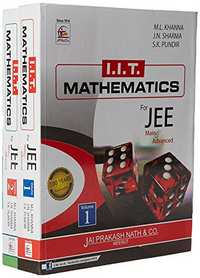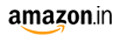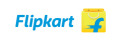Share

# IIT Mathematis For JEE (Main & Advanced) (Set Of 2 Volumes) (Paperback) JEE MainM.R.P 899/-1050.00-1175.00-
Product Description

Table of Contents Volume - I :- Section - I: Algebra Sets, Relations and Functions Complex Numbers Progressions Theory of Quadratic Equations Logarithms Miscellaneous Equations Permutations and Combinations Mathematical Induction Binomial Theorem Determinants Matrices Section - II: Trigonometry Trigonometrical Ratios and Identities Trigonometrical Equations Inverse Circular Functions Properties of Triangles Height and Distances Section - III: Co-ordinate Geometry The Straight Line (First Degree) The Circle Pair of Straight Lines Volume - II :- Section - IV: Co-ordinate Geometry The Parabola The Ellipse The Hyperbola Co-ordinate Geometry of Three Dimensions Section - V: Vector Algebra Addition of Vectors Multiplication of Vectors Section - VI: Differential Calculus Differentiation Functions Limits, Continuity and Differentiability Tangents and Normals Maxima and Minima Section - VII: Integral Calculus Integration Section - VIII: Differential Equations Differential Equations of 1st order and 1st degree, Formation of Differential Equations Section - IX: Statistics Measure of Central Tendency Section - X: Probability Probability Section - XI: Reasoning Mathematical Reasoning Fun in Mathematics IIT - JEE (Mains) Examination Paper 2013 IIT - JEE (Advanced) Examination Paper 2013